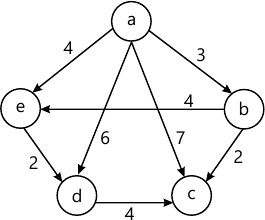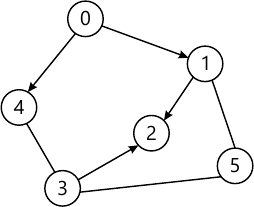MORE IN Data Structures & Algorithms
SPPU Electronics and Telecom Engineering (Semester 3)
Data Structures & Algorithms
December 2014
Total marks: --
Total time: --
INSTRUCTIONS
(1) Assume appropriate data and state your reasons
(2) Marks are given to the right of every question
(3) Draw neat diagrams wherever necessary

Answer any one question from Q1 and Q2
1 (a) Define recursion with example in detail.
4 M
1 (b) Write a function in 'C' to sort numbers using bubble sort.
4 M
1 (c) Differentiate between static memory and dynamic memory allocation.
4 M

2 (a) Write algorithm to add two polynomials using array of structures.
4 M
2 (b) Define pointers and write function 'swap' to swap two numbers using pointers.
4 M
2 (c) Explain bitwise operators with example in detail.
4 M

Answer any one question from Q3 and Q4
3 (a) Differentiate between SLL and DLL.
4 M
3 (b) Write function PUSH to implement stack using array.
4 M
3 (c) Name types of queues. Explain any one in detail.
4 M

4 (a) Write a function in 'C' to delete a node in SLL.
4 M
4 (b) Explain operation insert in linear queue.
4 M
4 (c) Convert the following infix expression to postfix using stacks:
a+b*(c/d \$ a)/b.
4 M

Answer any one question from Q5 and Q6
5 (a) Define BST. Create BST for the following numbers:
56, 34, 89, 11, 45, 67, 6, 78.
Show preorder traversal.
5 M
5 (b) Explain threaded binary tree with an example.
4 M
5 (c) Define the following terms with example:
(i) Strictly binary tree
(ii) Completely binary tree.
4 M

6 (a) Write a function in 'C' to search a number in BST.
5 M
6 (b) For the following numbers create AVL tree: 6 5 4 3 2 1.
4 M
6 (c) Explain expression trees with one example.
4 M

Answer any one question from Q7 and Q8
7 (a) Using Dijkstra's algorithm find out shortest path four source node (a) for the given graph:5 M
7 (b) Represent the given graph using adjacency matrix:4 M
7 (c) Explain Kruskal's algorithm with an example.
4 M

8 (a) Explain graph traversal methods with suitable example.
5 M
8 (b) Explain Prim's algorithm with suitable example.
4 M
8 (c) Define with example:
(i) Connected graph
(ii) Path.
4 M

More question papers from Data Structures & Algorithms